Home > CAAC > Chapter 7 > Lesson 7.1.5 > Problem7-48

7-48.
1. Consider this system of equations: Homework Help ✎

y = 2x − 8

y =1. Use your knowledge of y = mx + b to graph the lines without tables.

2. Use the graph to find the point of intersection.

3. Confirm this point of intersection by solving the system algebraically.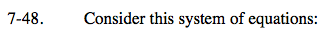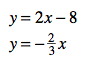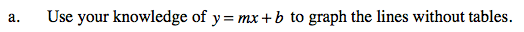Remember:
b = the y-intercept
m = the slope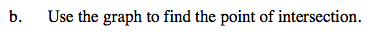The point of intersection is (3, −2). Where is this point on the graph?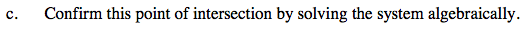Use the Equal Values Method.

Use the eTool below to help you solve this problem.
Click the link at right for the full version of the eTool: AC 7-48 HW eTool